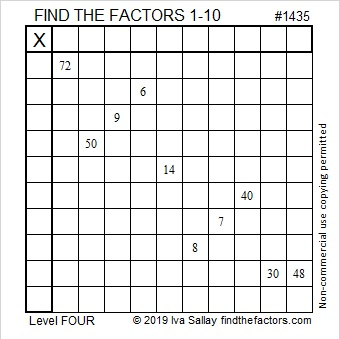# 1435 is the 23rd Friedman Number

41 ×35 = 1435. Since the same digits are used on both sides of the equal sign that makes 1435 the 23rd Friedman number.

Since it’s good for puzzles to have a puzzle number to distinguish them from each other, I’ve given this one the number 1435. Can you solve it?Print the puzzles or type the solution in this excel file:  10 Factors 1432-1442

Here are a few more facts about the number 1435:

• 1435 is a composite number.
• Prime factorization: 1435 = 5 × 7 × 41
• 1435 has no exponents greater than 1 in its prime factorization, so √1435 cannot be simplified.
• The exponents in the prime factorization are 1, 1, and 1. Adding one to each exponent and multiplying we get (1 + 1)(1 + 1)(1 + 1) = 2 × 2 × 2 = 8. Therefore 1435 has exactly 8 factors.
• The factors of 1435 are outlined with their factor pair partners in the graphic below.1435 is also the hypotenuse of FOUR Pythagorean triples:
315-1400-1435 which is 35 times (9-40-41)
588-1309-1435 which is 7 times (84-187-205)
861-1148-1435 which is (3-4-5) times 287
931-1092-1435 which is 7 times (133-156-205)